# Projectile Motion Worksheet

(A) A particle moves such a way that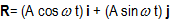where i and j are unit vectors along x and y direction. A and ω are constant
Question 1.
Find the trajectory of the particle
a. Circle
b. Parabola
c. Eclipse
d. None of the above

Question 2.
Find the Average velocity of the particle from t=0 to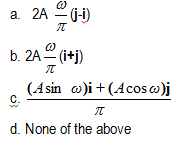Question 3.
Find the scalar Product of Acceleration and velocity at any point t
a. 1
b. -1
c. 0
d. None of the above

Question 4.
Find the tangential acceleration at any point of time t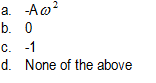Question 5.
Find the radial acceleration at any point of time t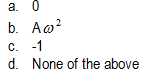Question 6.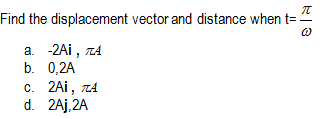(B) Two particles start from the origin of the horizontal x-y plane. Particle A moves along +x axis with uniform velocity u. Particle B moves along -y axis with velocity u. i and j are the unit vectors along x and y direction
Question 7.
Find the relative velocity of particle B w.r.t to A at time t
a. $-u(\mathbf{i}+\mathbf{j})$
b. $u(\mathbf{i}+\mathbf{j})$
c. $0$
d. none of the above

Question 8.
Find the relative velocity of particle A w.r.t to B at time t
a. $-u(\mathbf{i}+\mathbf{j})$
b. $u(\mathbf{i}+\mathbf{j})$
c. $0$
d. none of the above

Question 9.
find the relative position vector of particle A w.r.t to B at time t
a. $-ut(\mathbf{i}+\mathbf{j})$
b. $ut(\mathbf{i}+\mathbf{j})$
c. $0$
d. none of the above

## Subjective questions

Question 10.
A particle moves 4 km North East and reach position A and then moves 3 Km South East to reach the position B. Let assume the initial point has co-ordinates (0, 0).And Co-ordinates of the position A and B are
A -> (xa,ya)
B -> (xb,yb)
Also i and j are the unit vector across the x and y direction
Find out the following
a.Find out the co-ordinates of Position A and B
b.Find out the distance of point B from the Origin
c.Find out the position vector of point A and B

Question 11.
An object is fired upward at an angle 40° to the horizontal .The object is fired with an initial speed of 20m/s.
Find out the following
a. How high up will it strike a wall which is 8 m away
b. How much time it will take to strike the wall
c. What are the horizontal and vertical components of velocity when it strike the wall

Question 12.
The position of a particle is given by the below equation
$\mathbf{R}= (2sin2 \pi t)\mathbf{i} + (3cos2 \pi t)\mathbf{j}$
a. Find out the trajectory of the particle
b. Find out the velocity and acceleration vector and the relation between the acceleration and position vector.
c. Find out the times when velocity becomes maximum and minimum.
d. Find out the time dependence of the angle α between velocity and acceleration vector
e. Find the angle α at t=0 and t=1/4

Question 13.
A ball is thrown upward from a point O on the side of a hill which slopes upward uniformly at an angle 30°.Intial Velocity of the ball is $v_0$ and it is thrown at an angle 60° with respect to horizontal.
a. Find out the range along the slope of the hill
b. Find out the Time period of the Projectile
c. What height above the point O ,ball strike the incline plane
d. What velocity does the ball strike the plane

Question 14.
A particle moves in the plane xy with velocity given by $\mathbf{v}=a \mathbf{i}+bx \mathbf{j}$ where i  andj are the unit vectors of the x and y axis and a and b are constants. At the initial moment of time the particle was located at point x=y=0. Find
a. The equation of particle’s trajectory y(x)
b. The curvature radius of trajectory as a function of x.

Question 15.
A boy is standing at a distance $a_1$ from the foot of a tower. The boy throws an stone at a angle 45° which just touches the top of the tower and strikes the ground at a distance $a_2$ from the point the boy is standing. Find the height of the tower

Question 16.
A ball is thrown upward from a point on the side of a hill which slopes upward uniformly at angle 28°.Intial velocity of the ball is v0= 33 m/s and at an angle 65°(with respect to the horizontal. At what distance up the slope the ball strike and in what time?

Question 17.
A Cannon on a level plain is aimed at an angle θ above the horizontal. A shell is fired with a muzzle velocity v0 towards a pole which is distance R away. It hits the pole at height H.
a find the time taken to reach the pole
b. find the value of H in terms of θ,R and v0

Question 18.
The current velocity of a river grows in proportion to the distance from its bank and reaches its maximum v0 in the middle. The width of the river is b. The velocity near the banks is zero. A boat is so moving on the river that its velocity u relative to the water is constant and perpendicular to the current
a. Find the velocity of the current at a distance y from the bank ( y<b/2)
b. Find the velocity of the boat relative to ground at any point on it path ( y<b/2)
c. Find the velocity of the boat relative to ground at any point on it path ( b/2< y < b)
d. Find the acceleration also on both the above distances
e. Find the distance through which the boat crossing the river will be carried away by the current
f. Find the trajectory of the boat